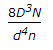# Civil Engineering - Theory of Structures

### Exercise :: Theory of Structures - Section 1

21.

In case of a simply supported rectangular beam of span L and loaded with a central load W, the length of elasto-plastic zone of the plastic hinge, is

 A.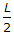B.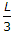C.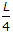D.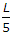E.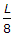Explanation:

No answer description available for this question. Let us discuss.

22.

Pick up the correct statement from the following:

 A. Hoop strain of the walls of a cylinder due to liquid is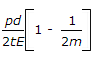B. Longitudinal strain in the walls of a cylinder due to liquid is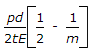C. Volumetric change in the cylinderdue to liquid is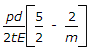D. All the above.

Explanation:

No answer description available for this question. Let us discuss.

23.

A material may fail if

 A. maximum principal stress exceeds the direct stress σ0 B. maximum strain exceeds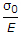C. maximum shear stress exceeds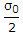D. total strain energy exceeds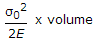E. all the above.

Explanation:

No answer description available for this question. Let us discuss.

24.

A truss containing j joints and m members, will be a simple truss if

 A. m = 2j - 3 B. j = 2m - 3 C. m = 3j - 2 D. j = 3m - 2

Explanation:

No answer description available for this question. Let us discuss.

25.

The stiffness of the close coil helical spring is

 A.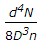B.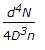C.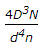D.